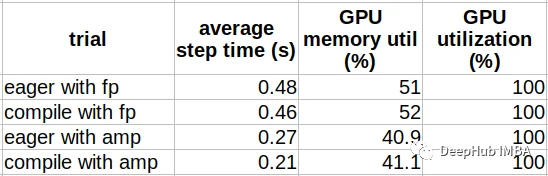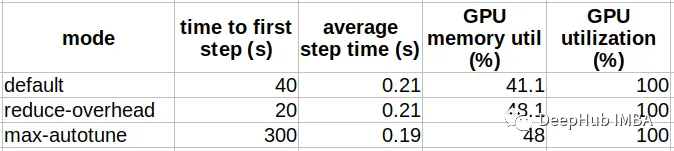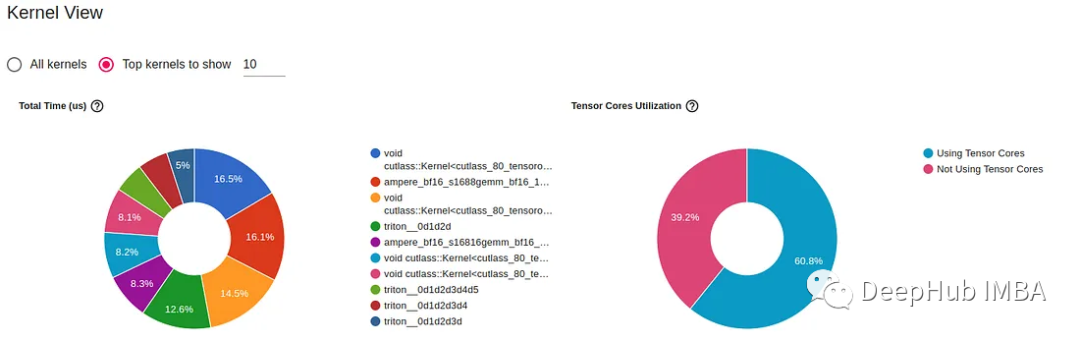PyTorch 2.0 发布也有一段时间了，大家是不是已经开始用了呢？ PyTorch 2.0 通过引入 torch.compile，可以显着提高训练和推理速度。 与 eagerly 模式相反，编译 API 将模型转换为中间计算图（FX graph），然后以某种方式将其编译为低级计算内核，这样可以提高运行速度。“只是用 torch.compile 调用包装它们就可以提高运行速度”

Torch 编译背后有许多创新技术，包括 TorchDynamo、FX Graph、TorchInductor、Triton 等。 我们不会在这篇文章中深入探讨不同的组件，如果你对这些感兴趣，可以查看PyTorch 文档，里面介绍的非常详细。

### TensorFlow 与 PyTorch 的两个不重要的对比

1、在过去，PyTorch 和 TensorFlow 之间有着明显的区别。 PyTorch 使用了 eager execution 模式，TensorFlow 使用了 graph 模式，大家都在各自发展。 但后来 TensorFlow 2 引入了eager execution作为默认执行模式，TensorFlow 变得有点像 PyTorch。 现在 PyTorch 也引入了自己的graph 模式解决方案，变得有点像 TensorFlow。 TensorFlow 与 PyTorch 的竞争仍在继续，但两者之间的差异正在慢慢消失。

2、人工智能开发是一个时髦的行业。 但是流行的 AI 模型、模型架构、学习算法、训练框架等随时间变化发展的。 就论文而言，几年前我们处理的大部分模型都是用 TensorFlow 编写的。 但是人们经常抱怨高级 model.fit API 限制了他们的开发灵活性，并且graph 模式使他们无法调试。 然后就有好多人转向了PyTorch，他们说，“PyTorch可以以任何想要的方式构建模型并轻松调试”。但是更灵活的的自定义操作会导致开发的复杂性，PyTorch Lightening等高级的API的出现就是复制了model.fit API的特性，然后同样的人又说还有人说“我们必须适应 PyTorch Lightening，我们必须用 torch.compile 加速我们的训练”。既要灵活，又要简单是不可能同时实现的。

### PyTorch3兼容性

PyTorch2的优点之一是它完全向后兼容，所以我们即使不使用torch.compile，仍然可以使用PyTorch 2.0并从其他新功能和增强中受益。最多就是享受不到速度的提升，但是不会有兼容性的问题。但是如果你想进一步提升速度，那么请往下看。

### 简单例子

`````` importtime, os
importtorch
fromtorch.utils.dataimportDataset
fromtimm.models.vision_transformerimportVisionTransformer

use_amp=True# toggle to enable/disable amp
use_compile=True# toggle to use eager/graph execution mode

# use a fake dataset (random data)
classFakeDataset(Dataset):
def__len__(self):
return1000000

def__getitem__(self, index):
rand_image=torch.randn([3, 224, 224], dtype=torch.float32)
label=torch.tensor(data=[index%1000], dtype=torch.int64)
returnrand_image, label

deftrain():
device=torch.cuda.current_device()
dataset=FakeDataset()
batch_size=64

# define an image classification model with a ViT backbone
model=VisionTransformer()

ifuse_compile:
model=torch.compile(model)

model.to(device)

batch_size=batch_size, num_workers=4)
loss_function=torch.nn.CrossEntropyLoss()

t0=time.perf_counter()
summ=0
count=0

inputs=inputs.to(device)
targets=torch.squeeze(target.to(device), -1)

withtorch.cuda.amp.autocast(
enabled=use_amp,
dtype=torch.bfloat16
):
outputs=model(inputs)
loss=loss_function(outputs, targets)

loss.backward()
optimizer.step()

batch_time=time.perf_counter() -t0

ifidx>10:  # skip first few steps
summ+=batch_time
count+=1
t0=time.perf_counter()
ifidx>500:
break

print(f'average step time: {summ/count}')

if__name__=='__main__':
train()
``````https://pytorch.org/get-started/pytorch-2.0/#distributed

### 高级选项

compile API包含许多用于控制graph创建的选项，能够针对特定模型对编译进行微调，并可能进一步提高性能。下面的代码块是官方的函数介绍：

`````` defcompile(model: Optional[Callable] =None, *,
fullgraph: builtins.bool=False,
dynamic: builtins.bool=False,
backend: Union[str, Callable] ="inductor",
mode: Union[str, None] =None,
options: Optional[Dict[str, Union[str, builtins.int, builtins.bool]]] =None,
disable: builtins.bool=False) ->Callable:
"""
Optimizes given model/function using TorchDynamo and specified backend.

Args:
model (Callable): Module/function to optimize
fullgraph (bool): Whether it is ok to break model into several subgraphs
dynamic (bool): Use dynamic shape tracing
backend (str or Callable): backend to be used
mode (str): Can be either "default", "reduce-overhead" or "max-autotune"
options (dict): A dictionary of options to pass to the backend.
disable (bool): Turn torch.compile() into a no-op for testing
"""
``````backend 编译器后端:API使用哪个后端将中间表示(IR)计算图(FX graph)转换为低级内核操作。这个选项对于调试graph编译问题和更好地理解torch.compile的内部非常有用。在大多数情况下，默认的Inductor后端似乎能够提供最佳的训练性能结果。有很多后端列表，我们可以使用下面命令查看：

`````` fromtorchimport_dynamo
print(_dynamo.list_backends())
``````

fullgraph 强制单个图:这个参数是非常有用，可以确保没有任何不希望的图截断。

dynamic 动态形状:目前 2.0对具有动态形状的张量的编译支持在某种程度上是有限的。编译具有动态形状的模型的一个常见解决方案是重新编译，但会大大增加开销并大大降低训练速度。如果您的模型确实包含动态形状，将动态标志设置为True将带来更好的性能，特别是减少重新编译的次数。

### 性能分析

PyTorch Profiler是用来分析PyTorch模型性能的关键工具之一，可以评估和分析图编译优化训练步骤的方式。在下面的代码块中，我们用profiler生成TensorBoard的结果，来查看训练的性能：

``````   out_path=os.path.join(os.environ.get('SM_MODEL_DIR','/tmp'),'profile')
fromtorch.profilerimportprofile, ProfilerActivity
withprofile(
activities=[ProfilerActivity.CPU, ProfilerActivity.CUDA],
schedule=torch.profiler.schedule(
wait=20,
warmup=5,
active=10,
repeat=1),
dir_name=out_path)

) asp:
inputs=inputs.to(device)
targets=torch.squeeze(target.to(device), -1)

withtorch.cuda.amp.autocast(
enabled=use_amp,
dtype=torch.bfloat16
):
outputs=model(inputs)
loss=loss_function(outputs, targets)
loss.backward()
optimizer.step()
p.step()
``````### 调试模型编译问题

torch.compile 目前处于测试阶段，如果你遇到问题，并且幸运的话，会得到一个信息错误，我们可以直接搜索解决，或者问问chatgpt。 但是如果你不那么幸运，就需要自己寻找问题的根源。

`````` importos, logging
importtorch
fromtorchimport_dynamo

# enable debug prints
torch._dynamo.config.log_level=logging.INFO
torch._dynamo.config.verbose=True

# uncomment to run minifier
# torch._dynamo.config.repro_after="aot"

defbuild_model():
importtorch.nnasnn
importtorch.nn.functionalasF

classDumbNet(nn.Module):
def__init__(self):
super().__init__()
self.conv1=nn.Conv2d(3, 6, 5)
self.pool=nn.MaxPool2d(2, 2)
self.fc1=nn.Linear(1176, 10)

defforward(self, x):
x=self.pool(F.relu(self.conv1(x)))
x=torch.flatten(x, 1)
x=self.fc1(x)
sum_vals=torch.sum(x,0)
# this is the problematic line of code
torch.distributed.all_reduce(sum_vals)
x=x+0.1*sum_vals
returnx

net=DumbNet()
returnnet

deftrain():
'localhost')
os.environ['MASTER_PORT'] =os.environ.get('MASTER_PORT',
str(2222))
torch.distributed.init_process_group('nccl', rank=0,
world_size=1)
torch.cuda.set_device(0)
device=torch.cuda.current_device()

model=build_model()

model=torch.compile(model)

# replace with this to verfiy that error is not in TorchDynamo
# model = torch.compile(model, 'eager')
# replace with this to verfiy that error is not in AOTAutograd
# model = torch.compile(model, 'aot_eager')

model.to(device)

rand_image=torch.randn([4, 3, 32, 32], dtype=torch.float32).to(device)

model(rand_image)

if__name__=='__main__':
train()
``````

### 常见的图截断问题

Pytorch eager 模式优势之一是能够将纯 Pythonic 代码与 PyTorch 操作交织在一起。 但是这种自由在使用 torch.compile 时受到很大限制。因为 Pythonic 操作导致 TorchDynamo 将计算图拆分为多个组件，从而阻碍了性能提升的潜力。 而我们代码优化的目标是尽可能减少此类图截断。 最简单的办法是用 fullgraph 标志编译模型。这杨可以提示删除导致图截断的任何代码，而且还会告诉我们如何最好地适应PyTorch2的开发习惯。 但是要运行分布式代码，则必须将他设为False，因为当前实现 GPU 之间通信的方式需要图拆分。 我们也可以使用 torch._dynamo.explain 序来分析图截断。

`````` importtorch
fromtorchimport_dynamo
importnumpyasnp

defbuild_model():
importtorch.nnasnn
importtorch.nn.functionalasF

classDumbNet(nn.Module):
def__init__(self):
super().__init__()
self.conv1=nn.Conv2d(3, 6, 5)
self.pool=nn.MaxPool2d(2, 2)
self.fc1=nn.Linear(1176, 10)
self.fc2=nn.Linear(10, 10)
self.fc3=nn.Linear(10, 10)
self.fc4=nn.Linear(10, 10)
self.d= {}

defforward(self, x):
x=self.pool(F.relu(self.conv1(x)))
x=torch.flatten(x, 1)
asserttorch.all(x>=0) # graph break
x=self.fc1(x)
self.d['fc1-out'] =x.sum().item() # graph break
x=self.fc2(x)
forkinnp.arange(1): # graph break
x=self.fc3(x)
print(x)  # graph break
x=self.fc4(x)
returnx

net=DumbNet()
returnnet

deftrain():
model=build_model()
rand_image=torch.randn([4, 3, 32, 32], dtype=torch.float32)
explanation=torch._dynamo.explain(model, rand_image)
print(explanation)

if__name__=='__main__':
train()
``````

### 训练问题故障排除

graph模式下的调试比 eager 模式下的调试困难得多。 在 eager 模式下，每一行代码都是独立执行的，我们可以在代码中的任意点放置断点获得前张量值。 而在graph 模式下，代码定义的模型在处理之前会经历多次转换，设置的断点可能不会被触发。

### 在图中包含损失函数

`````` importtorch
fromtimm.models.vision_transformerimportVisionTransformer

classExpensiveLoss(torch.nn.Module):
def__init__(self):
super(ExpensiveLoss, self).__init__()
self.expert_model=VisionTransformer(depth=24)
iftorch.cuda.is_available():
self.expert_model.to(torch.cuda.current_device())
self.mse_loss=torch.nn.MSELoss()

defforward(self, input, outputs):
expert_output=self.expert_model(input)
returnself.mse_loss(outputs, expert_output)
``````

1、loss函数封装在torch.compile调用中，如下所示:

`````` loss_function=ExpensiveLoss()
compiled_loss=torch.compile(loss_function)
``````

2、创建一个包含模型和损失的包装器模型来将模型和损失一起编译，并将结果损失作为输出返回。

`````` importtime, os
importtorch
fromtorch.utils.dataimportDataset
fromtorchimportnn
fromtimm.models.vision_transformerimportVisionTransformer

# use a fake dataset (random data)
classFakeDataset(Dataset):
def__len__(self):
return1000000

def__getitem__(self, index):
rand_image=torch.randn([3, 224, 224], dtype=torch.float32)
label=torch.tensor(data=[index%1000], dtype=torch.int64)
returnrand_image, label

# create a wrapper model for the ViT model and loss
classSuperModel(torch.nn.Module):
def__init__(self):
super(SuperModel, self).__init__()
self.model=VisionTransformer()
self.expert_model=VisionTransformer(depth=24iftorch.cuda.is_available() else2)
self.mse_loss=torch.nn.MSELoss()

defforward(self, inputs):
outputs=self.model(inputs)
expert_output=self.expert_model(inputs)
returnself.mse_loss(outputs, expert_output)

# a loss that simply passes through the model output
classPassthroughLoss(nn.Module):
def__call__(self, model_output):
returnmodel_output

deftrain():
device=torch.cuda.current_device()
dataset=FakeDataset()
batch_size=64

# create and compile the model
model=SuperModel()
model=torch.compile(model)

model.to(device)

batch_size=batch_size, num_workers=4)

loss_function=PassthroughLoss()

t0=time.perf_counter()
summ=0
count=0

inputs=inputs.to(device)
targets=torch.squeeze(target.to(device), -1)

withtorch.cuda.amp.autocast(
enabled=True,
dtype=torch.bfloat16
):
outputs=model(inputs)
loss=loss_function(outputs)

loss.backward()
optimizer.step()

batch_time=time.perf_counter() -t0

ifidx>10:  # skip first few steps
summ+=batch_time
count+=1
t0=time.perf_counter()
ifidx>500:
break

print(f'average step time: {summ/count}')

if__name__=='__main__':
train()
``````

### 总结

PyTorch 2.0编译模式具有显著提高训练和推理速度的潜力，可以显著节省成本，但是模型实现这一潜力所需的工作量可能会有很大差异。许多公共模型只需要修改一行代码。而其他模型特别是那些包含非标准操作、动态形状和/或大量交错Python代码的模型，可能得不偿失甚至无法进行。但是现在开始修改模型是一个很好的选择，因为目前来看torch.compile对于PyTorch2来说是一个重要且持续的特性。

https://avoid.overfit.cn/post/dfea563957fc43a19f1aaf7733888031